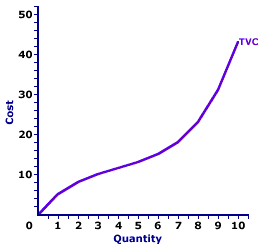Tuesday  May 30, 2023
 AmosWEB means Economics with a Touch of Whimsy!REPURCHASE AGREEMENT: A common type of bank account in which funds are transferred from one account to another, then automatically transferred back after a short period, usually overnight. In effect, a bank customer buys a legal claim from a bank with the understanding that the bank will automatically "repurchase" this legal claim back after a specified time period. Repurchase agreements were original developed as a round about means of paying interest on business checking, which such interest paying was legally prohibited. Repurchase agreements are near monies added to M1 to obtain broader monetary aggregates, M2 and M3.TOTAL VARIABLE COST CURVE:

A curve that graphically represents the relation between total variable cost incurred by a firm in the short-run production of a good or service and the quantity produced. When constructing this curve, it is assumed that total variable cost changes as a result of changes in the quantity of output produced, while other variables like technology and resource prices are held fixed. The total variable cost curve is one of three total cost curves, the other two are total cost curve and total fixed cost curve.
Total Variable Cost CurveThe total variable cost curve illustrates the graphical relation between total variable cost and the quantity of output produced. The shape of the total variable cost curve reflects increasing marginal returns at small quantities of output and decreasing marginal returns at large quantities. The marginal cost curve, THE focal point for the analysis of short-run production, can be derived directly from the total variable cost curve.

The total variable cost curve can be derived in two ways. One is to plot a schedule of numbers relating output quantity and total variable cost. The other is to vertically subtract the total fixed cost curve from the total cost curve. The slope of this total variable cost curve is marginal cost.

The graph to the right is the total variable cost curve for the short-run production of Wacky Willy Stuffed Amigos (those cute and cuddly armadillos and tarantulas). The quantity of Stuffed Amigos production, measured on the horizontal axis, ranges from 0 to 10 and the total cost incurred in the production of Stuffed Amigos, measured on the vertical axis, ranges from \$0 to \$43.

The most striking feature of the total variable cost curve is its shape. The total variable cost curve emerges from the origin, then twists and turns its way to \$43. This curve begins relatively steep, then flattens, before turning increasingly steep once again. In fact, it is somewhat reminiscent of the total product curve. As a mater of fact, the total variable cost curve can be derived directly from the total product curve.

The slope of the total variable cost curve flattens as the first four Stuffed Amigos are produced. This range of output corresponds with increasing marginal returns found in Stage I of production. Increasing marginal returns causes the total variable cost curve to flatten. The slope of the total variable cost curve becomes increasingly steeper after the fourth Stuffed Amigo is produced. This range of output corresponds with decreasing marginal returns, and the extremely important law of diminishing marginal returns, found in Stage II of production. Deceasing marginal returns causes the total variable cost curve to become steeper.

The total variable cost curve provides the foundation upon which the total cost curve is built. In fact, the total cost curve and the total variable cost curve are parallel, matching slope for slope at each quantity, with the equal distance being total fixed cost. The equality of the slopes means that both curves can be used to derive marginal cost. The total variable cost curve is also important as the basis for deriving the average variable cost curve.

 <= TOTAL VARIABLE COST AND TOTAL PRODUCT TRADE BARRIERS =>Recommended Citation:

TOTAL VARIABLE COST CURVE, AmosWEB Encyclonomic WEB*pedia, http://www.AmosWEB.com, AmosWEB LLC, 2000-2023. [Accessed: May 30, 2023].

Check Out These Related Terms...

Or For A Little Background...

And For Further Study...
Search Again?ORANGE REBELOON[What's This?] Today, you are likely to spend a great deal of time looking for a downtown retail store wanting to buy either decorative picture frames or storage boxes for your income tax returns. Be on the lookout for celebrities who speak directly to you through your television.Your Complete ScopeA scripophilist is one who collects rare stock and bond certificates, usually from extinct companies."Never let the fear of striking out get in your way. "-- Babe RuthICCInternational Chamber of CommerceA PEDestrian's Guide Xtra CreditTell us what you think about AmosWEB. Like what you see? Have suggestions for improvements? Let us know. Click the User Feedback link.| | | | | | | | | | |
| | | |

Thanks for visiting AmosWEB